Designing a logo is not that simple as it seems. It all depends on a designer that he/she should create a brand’s visual identity in an attractive way, not just the simple text.

When a viewer knows what the company does by just seeing their logo then, we can say the work of the designer is full-filled. A designer creates a logo using some techniques. We are presenting here basic techniques that help you to design a logo that will be up to the mark and grabs the attention of all. It is called the GOLDEN RATIO technique.

What is GOLDEN RATIO and how to use it?

Golden Ratio is a basic rule of all types of design. While designing anything, the designer would consider this mathematical rule in his/her mind. Golden Ratio is found in our nature and it has been created from there as well. Every beautiful thing that we see in nature has made up of golden ratio.

Now let’s talk about how a Golden Ratio works and how we can apply it in our designs. It can be understood by the Fibonacci series. Fibonacci was a famous researcher who founded the Golden Ratio rule first and developed a series of numbers called the Fibonacci series. This series starts from 0 and goes to infinite. As we go along, we add our last no. to the previous no. to make the third no.

For example – Assume the first no. is 0. Now if we add the next no. 1 into it, we find our third no. which is 1. Next, we add 1 into 1 and find the next third no. 2…..and so on.

0 , 1 , 1 , 2 , 3 , 5 , 8 , 13 , 21 , 34 , 55 , 89…….. goes to infinite.

When we subtract our bigger no. from the lower no. , we will always get 1.618 as an answer. Like 8/5 = 1.618 , 5/3 = 1.618 , 3/2 = 1.618 and so on.

This can be easily understand by such diagrams below –

1. Take a square of 1:1 ( x=1 , y=1 )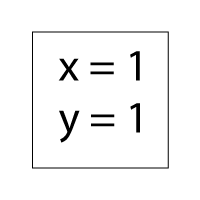1. Multiply 1 side of this square by 1.618 and you will get a rectangle like shape. (x=1.618 , y=1)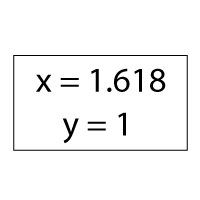1. Now, lay the square over the rectangle , and the two shapes will give you the Golden Ratio :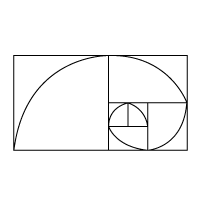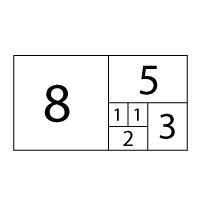How to use Golden Ratio in Logo Design?

When you know the concept of the Golden Ratio, it is easy for you to create it on your design. Divide your design area into a ratio of 1:1.618. That means if we assume the width from x and height from y, x is 1.618 and y is

1 . You designing area will look like this :-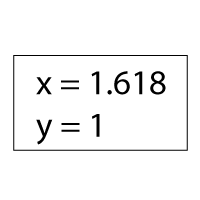Your area has now a Golden Ratio in it.Make an arc in each square and you will see the Golden Ratio shape in it.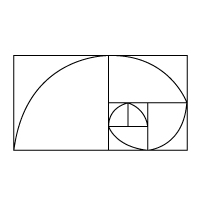Here you are getting ready to make a wonderful Logo Design by using this basic technique called “The Golden Ratio Technique”.

Categories: Graphic Design